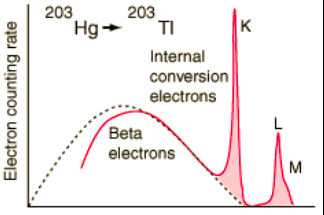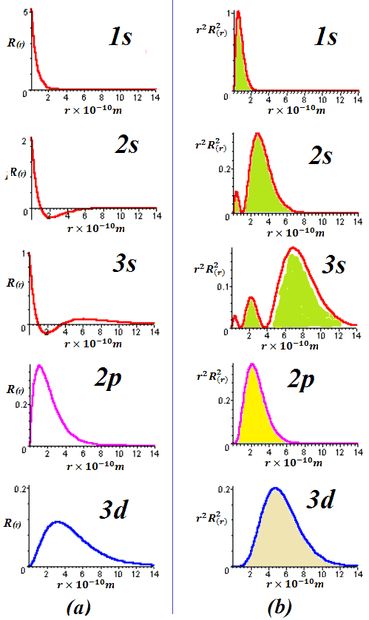# Electrons from Internal Conversion

• I
• Zuzana
The radius of the nucleus that undergo internal conversion is smaller for excited states (e.g. L-shell i.e. 2s, 2p) than for the ground state (K-shell i.e. 1s).f

#### Zuzana

Hi,
I would like to ask, why K-shell electrons coming from the internal conversion are much more frequent than L or M-shell electrons (see Fig). K-shell electrons are more tightly bound than L-shell, I would say that it is easier for gamma particle to kick off less tightly electron, no?The probability of an electron being found within the nucleus decreases as the shell increases. It is more probable for a K electron to be involved in the internal conversion process than an L or M electron.

•Zuzana and Astronuc
The probability of an electron being found within the nucleus decreases as the shell increases. It is more probable for a K electron to be involved in the internal conversion process than an L or M electron.
what do you mean by "within the nucleus"? electrons are at the atomic orbitals (shells outside the nucleus).

what do you mean by "within the nucleus"? electrons are at the atomic orbitals (shells outside the nucleus).
No, the wave function is extended. There is a non-zero probability of finding the electron at the nucleus.•vanhees71, PeroK and Zuzana
Aha, DrClaude beat me to it while I was Googling. Here's another set of examples:

http://hyperphysics.phy-astr.gsu.edu/hbase/hydwf.html

The probability of the electron having a small-enough value of ##r## to be inside the nucleus, is smaller for excited states (e.g. L-shell i.e. 2s, 2p) than for the ground state (K-shell i.e. 1s).

•vanhees71 and Zuzana
Now, I understand. Thanks a lot for the reply.

•berkeman
On the graphs at the link that I posted, the horizontal axis is given as multiples of ##a_0##, the Bohr radius (the radius of the K shell in the Bohr model).

Exercise: look up typical values for the radius of nuclei that undergo internal conversion, and compare to ##a_0##, so as to see what part of those graphs comes into play in internal conversion.